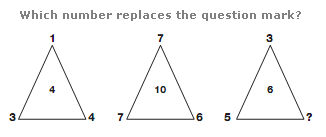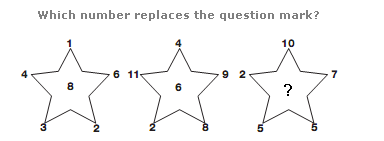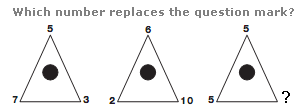# Puzzles - Number puzzles

Exercise : Number puzzles
28.:
4
Explanation
:
In each triangle, the central number equals the sum of the three outer numbers divided by two.

29.:
5
Explanation
:
In each star, the central number equals the difference between the sum of the even numbers and the sum of the odd numbers from the points of the star.

30.